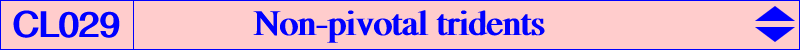See CL063 and CL070 for other kinds of circum-tridents which are psKs. *** For any point X = u : v : w on the line at infinity, the barycentric square W of X lies on the inscribed Steiner ellipse. R is the intersection of the trilinear polar of X (which is the tangent at W to the inscribed Steiner ellipse) and the polar line of X in the Steiner circum-ellipse. R = (v-w)u^2 : (w-u)v^2 : (u-v)w^2 is a point on K219. The tripolar centroid TG(X) of X is defined in Clark Kimberling's ETC, preamble before X(1635). For any point X different of G, TG(X) is in fact the isobarycenter of the three points of intersection of the trilinear polar of X with the sidelines of ABC. When X = u : v : w, we have TG(X) = u(v-w)(v+w-2u) : : , and when X lies at infinity, we obtain R as above. The cubic nK0(W, R) = cK0(#X, R) is a trident with point at infinity X which is a flex. Its equation is : (v-w)u^2 x(w^2y^2+v^2z^2) + cyclic = 0. For example, K218 is the trident with asymptote perpendicular to the Euler line. See also CL030 and a generalization in the page P-conical cubics.The real asymptote is the line XW meeting the trilinear polar of R at Z = (v-w)^2u^2 : (w-u)^2v^2 : (u-v)^2w^2 on the inscribed Steiner ellipse. Z is the center of the circum-conic passing through G and X. The tangent at Z to the inscribed Steiner ellipse passes through R. In other words, the asymptote is the polar line of the root R in the inscribed Steiner ellipse. This asymptote has equation : vw(v-w)x + cyclic = 0. The parabola asymptote has equation : u^2(w-u)(u-v)[vwx^2 - 2(u^2 - 2vw)yz] + cyclic = 0. The tangent at W to the inscribed Steiner ellipse meets the parallel at G to the real asymptote at Y on the curve. This trident is obviously a conico-pivotal cubic or cK and the pivotal conic is a parabola tangent to the real asymptote and inscribed in the anticevian triangle of X. See Special Isocubics §8.More generally, for X given on the line at infinity, one can find infinitely many other tridents which are cK(#X, P). It is sufficient to take P on the trilinear polar of the isotomic conjugate of X. For example, if X = X(523), P must lie on the line X(2)-X(6) giving in particular K218 (P = X1648) and K1072 (P = X6) . Conversely, for a given root P = u : v : w ≠ X(2), there is one and only one cK which is a trident (K). The singular point at infinity F must be the infinite point of the trilinear polar of tP hence F = v - w : w - u : u - v. The trident is then cK(#F, P) and the parabolic asymptote (P) has equation : ∑ (u + v - w) (u - v + w) x^2 + 2 [2(v - w)^2 + u (-u + v + w)] y z = 0. (K) has another asymptote (A) which is the trilinear polar of taP, a line with infinite point F tangent to the pivotal conic (a parabola) at Q which also lies on (P). Note that the tangent at Q to (P) contains the three finite points of inflexion of (K) but one only is real. (K) becomes a cK0 when P lies on K219 as seen above.